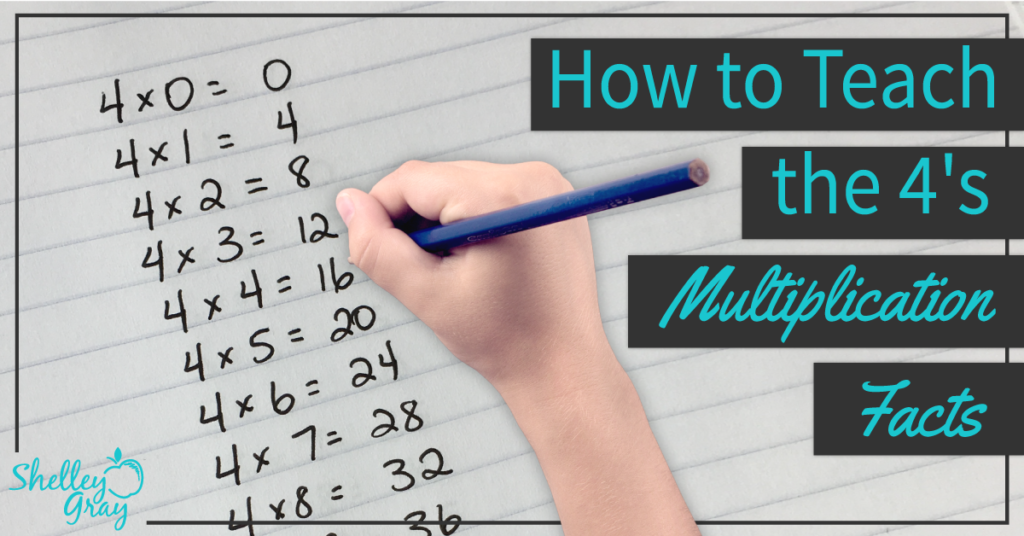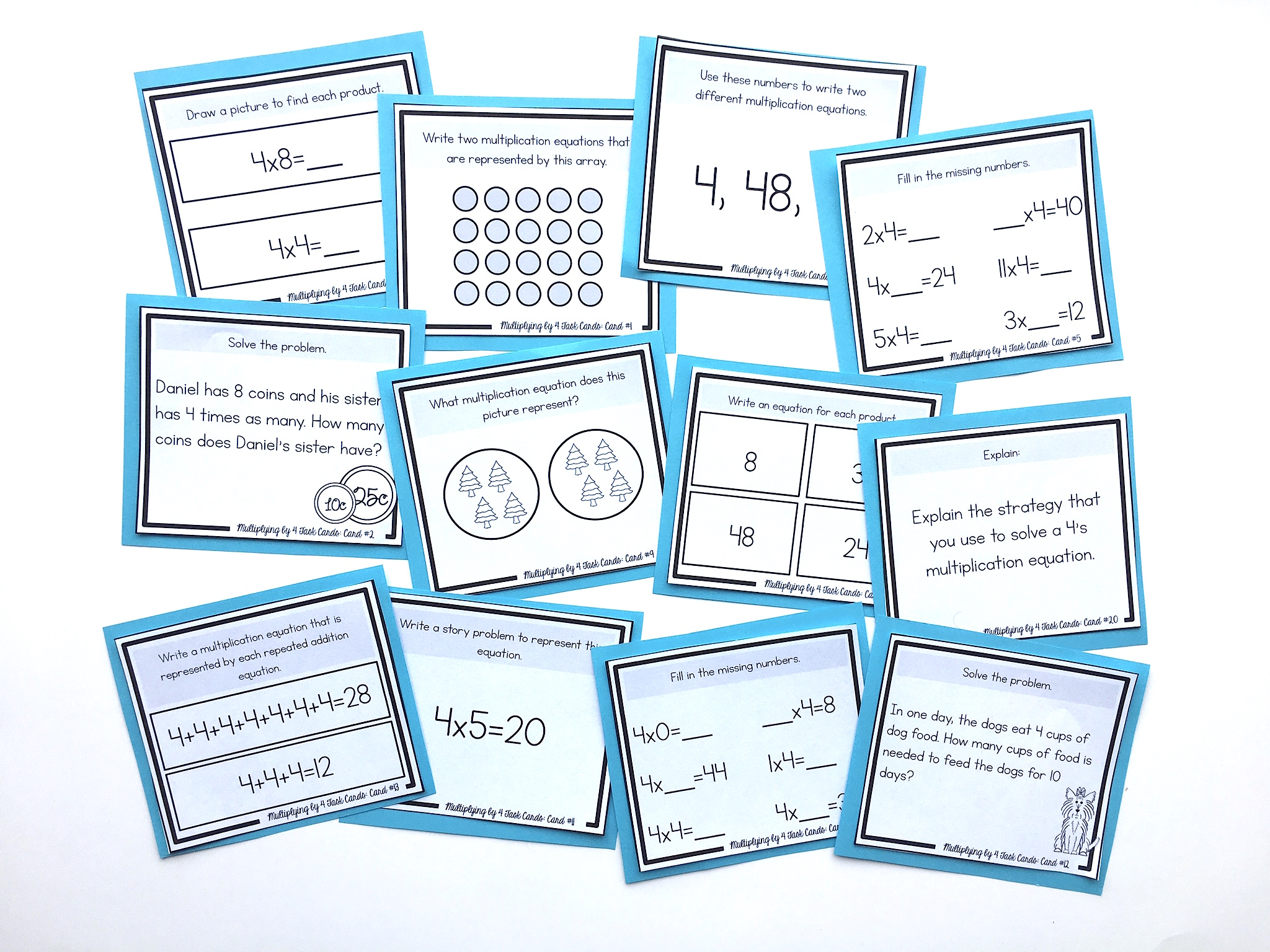# How to Teach the 4 Times Table So It Really Makes SenseThe 4’s multiplication facts are typically an easy set of facts to learn. This is because there is a specific strategy for this set of facts that is very effective.

The 4’s facts can be thought of as the doubles’ doubles.

For example, when you have an equation like 4×5, you can double the 5 to make 10, and then double the 10 to make 20.

For 4×6, find the double of 6 (12), and then double the 12 to make 24.

It’s also very important to reinforce the commutative property.

This means that the order of factors does not change the product. For example, if students are faced with an equation like 7×4, they should see the 4 as one of the factors, and know that they can use the 4’s strategy to solve this equation. In this case students can think, “the double of 7 is 14, and the double of 14 is 28.”

NEXT STEPS

Reinforce the 4’s multiplication facts with this set of task cards. Students will learn conceptually through problem-solving, using arrays, strategic thinking, finding missing numbers, skip-counting, picture representations, and more:OR FIND THE FULL BUNDLE OF MULTIPLICATION TASK CARDS HERE.